# AP Physics B : Momentum

## Example Questions

### Example Question #1 : Understanding Conservation Of Momentum

A baseball pitcher throws a baseball at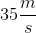horizontally in the positive direction to a batter, who hits the ball in the opposite direction at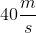. What is the change in momentum of the baseball if the baseball has a mass of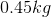?

Possible Answers: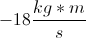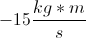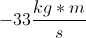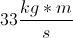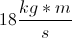Correct answer:Explanation:

In order to calculate the change in momentum, we must find the initial and final momentum of the baseball, and then find the difference.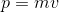Use the given velocities and mass to calculate the initial and final values.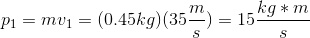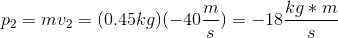The initial momentum is positive because the problem states that the ball was originally thrown in the positive direction. The final momentum is negative due to the change in direction.

Now we find the change in momentum: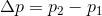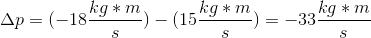### Example Question #14 : Translational Motion

Ball A, traveling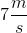to the right, collides with ball B, traveling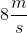to the left. If ball A is 4kg and ball B is 6kg, what will be the final velocity and direction after a perfectly inelastic collision?

Possible Answers: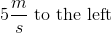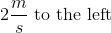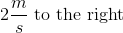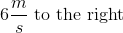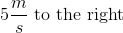Correct answer:Explanation:

A perfectly inelastic collision is when the two bodies stick together at the end. At the beginning the two balls are traveling separately with individual momentum values. Using the momentum equation, we can see that ball A has a momentum of (4kg)(7m/s) to the right and ball B has a momentum of (6kg)(8m/s) to the left. The final momentum would be the mass of both balls times the final velocity, (4+6)(vf). We can solve for vf through conservation of momentum; the sum of the initial momentum values must equal the final momentum.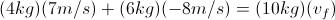Note: ball B's velocity is negative because they are traveling in opposite directions.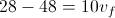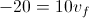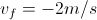The negative sign indicates the direction in which the two balls are traveling. Since the sign is negative and we indicated that traveling to the left is negative, the two balls must be traveling 2m/s to the left after the perfectly inelastic collision.

### Example Question #4 : Newtonian Mechanics And Motion

Two astronauts, Ann and Bob, conduct a collision experiment in a weightless, frictionless environment. Initially Ann moves to the right with a momentum of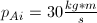, and Bob is initially at rest. In the collision, the two astronauts push on each other so that Ann's final momentum is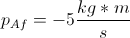to the left. What is Bob's final momentum?

Possible Answers: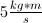to the right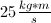to the leftto the rightto the left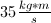to the right

Correct answer:to the right

Explanation:

Apply conservation of momentum before and after the collision.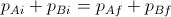.

Taking left to be the negative direction, and noting that Bob's initial momentum is 0 since he is at rest, we can use the provided information to see that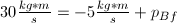.

Solving for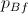, we get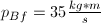. Since this answer is positive, Bob's momentum is in the positive direction (to the right).

### Example Question #1 : Momentum

A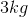mass traveling to the right on a two-dimensional Cartesian plane at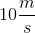, on a path inclined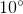to the positive x-axis, collides and sticks to a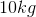mass traveling to the left directly along the x-axis (no inclination) at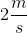.  What is the velocity and direction, with respect to the x-axis, of the combined body after the collision?

Possible Answers: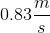at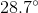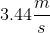at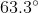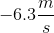at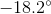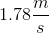at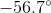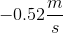at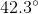Correct answer:atExplanation:

This is a completely inelastic collision, and, as with all collisions, it conserves linear momentum. To get magnitude and direction in two dimensions, it is typically best to look at the horizontal and vertical directions individually.

Horizontal direction: Themass is moving to the right at an inclination to the x-axis of. The horizontal component of its momentum is: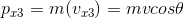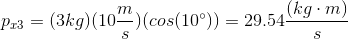Themass is moving exactly to the left (no inclination), so its momentum is all in the horizontal direction, but with a negative sign to indicate moving to the left on the Cartesian axes.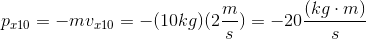So the total x-momentum after the collision is the sum of these two initial x-momenta, since total momentum is conserved in the collision.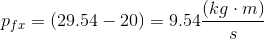This immediately shows that the combined mass after the collision is moving in the positive x-direction.

We now need to compute the final velocity in the x-direction. In this case: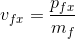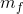is the total combined mass of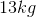after the collision, and we know the final momentum, allowing us to solve for the final horizontal velocity.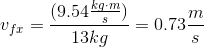Vertical direction: The same procedure can be applied to the y-direction. In this case, the process is simpler, because only themass contributes momentum in the y-direction, and that momentum will be the same before and after the collision.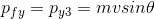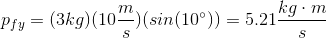Since the final momentum is now known to be positive in both the horizontal and vertical directions, the angle with respect to the x-axis must be positive. Now, the final y-direction velocity can be calculated.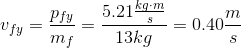Final velocity: With the final velocity components known, the final velocity can be calculated from trigonometry.

Magnitude: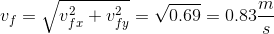Direction: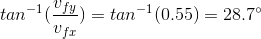### All AP Physics B Resources